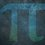# Number Spy Challenge $1$

Find a number that when divided by the Hardy-Ramanujan taxicab number $1$, it produces a number such that the sum of it's digits is equivalent to a quarter of a power of $2$.

In simplified terms:

$\frac{x}{1729}$ $= abcd$ such that $a + b + c + d =$$\frac{2^n}{4}$

The only condition is that $x \geq 1729$ as $\frac{1729}{1729}$ $= 1$.

You're looking for the first number.Note by Yajat Shamji
11 months, 3 weeks ago

This discussion board is a place to discuss our Daily Challenges and the math and science related to those challenges. Explanations are more than just a solution — they should explain the steps and thinking strategies that you used to obtain the solution. Comments should further the discussion of math and science.

When posting on Brilliant:

• Use the emojis to react to an explanation, whether you're congratulating a job well done , or just really confused .
• Ask specific questions about the challenge or the steps in somebody's explanation. Well-posed questions can add a lot to the discussion, but posting "I don't understand!" doesn't help anyone.
• Try to contribute something new to the discussion, whether it is an extension, generalization or other idea related to the challenge.
• Stay on topic — we're all here to learn more about math and science, not to hear about your favorite get-rich-quick scheme or current world events.

MarkdownAppears as
*italics* or _italics_ italics
**bold** or __bold__ bold
- bulleted- list
• bulleted
• list
1. numbered2. list
1. numbered
2. list
Note: you must add a full line of space before and after lists for them to show up correctly
paragraph 1paragraph 2

paragraph 1

paragraph 2

[example link](https://brilliant.org)example link
> This is a quote
This is a quote
    # I indented these lines
# 4 spaces, and now they show
# up as a code block.

print "hello world"
# I indented these lines
# 4 spaces, and now they show
# up as a code block.

print "hello world"
MathAppears as
Remember to wrap math in $$ ... $$ or $ ... $ to ensure proper formatting.
2 \times 3 $2 \times 3$
2^{34} $2^{34}$
a_{i-1} $a_{i-1}$
\frac{2}{3} $\frac{2}{3}$
\sqrt{2} $\sqrt{2}$
\sum_{i=1}^3 $\sum_{i=1}^3$
\sin \theta $\sin \theta$
\boxed{123} $\boxed{123}$

## Comments

Sort by:

Top Newest
- 11 months, 3 weeks ago

Log in to reply

@Yajat Shamji- Is $abcd=a\times b\times c \times d$ or they are digits?

- 11 months, 3 weeks ago

Log in to reply

I think $abcd$ is the four-digit number. Yes, I agree that it might be confusing because the result can be more than a four-digit number, but let him clarify

- 11 months, 3 weeks ago

Log in to reply

- 11 months, 3 weeks ago

Log in to reply

As $x$ can be equal to $1729$ ($\because x\geq1729$). If $x=1729$ then $a=0,b=0,c=0,d=1;a+b+c+d=1=\dfrac{2^2}{4}$ therefore $x=1729$

- 11 months, 3 weeks ago

Log in to reply

$\dfrac{1729}{1729} = 0001 = 0 + 0 + 0 + 1 = 1 = \dfrac{2^2}{4}$

There might be infinite of these

- 11 months, 3 weeks ago

Log in to reply

It is written that $\dfrac{x}{1729}=abcd;a+b+c+d=\dfrac{2^n}{4}$ not $\red{\dfrac{x}{1729}=\dfrac{2^n}{4}}$

- 11 months, 3 weeks ago

Log in to reply

What is asked is the sum of digits after the division and that is 1

- 11 months, 3 weeks ago

Log in to reply

thanks!

- 11 months, 3 weeks ago

Log in to reply

No. What is asked is that the sum of the digits equals a quarter of a power of $2$.

- 11 months, 2 weeks ago

Log in to reply

Thanks for finding the first number, @Mahdi Raza, @Zakir Husain!

- 11 months, 2 weeks ago

Log in to reply

@Mahdi Raza, @Zakir Husain - I am posting Number Spy Challenge $2$ in $\leq 5$ minutes! After that, I'll see you tomorrow!

- 11 months, 2 weeks ago

Log in to reply

×

Problem Loading...

Note Loading...

Set Loading...